# Harney & Sons Tea

The finest quality tea bags with a variety of wonderful blends!

Showing 1 - 12 of 20

•Quantity Decrease Quantity Increase Quantity
•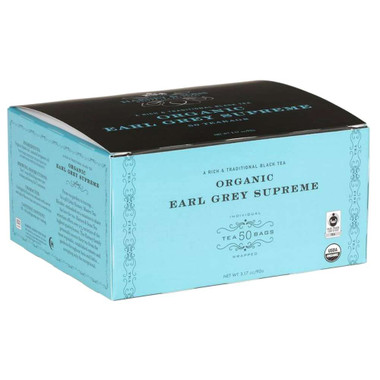Quantity Decrease Quantity Increase Quantity
•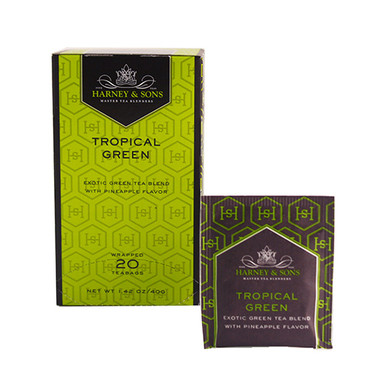Quantity Decrease Quantity Increase Quantity
•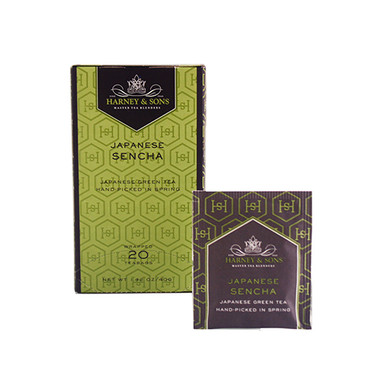Quantity Decrease Quantity Increase Quantity
•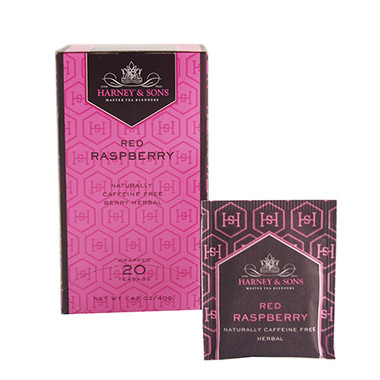Quantity Decrease Quantity Increase Quantity
•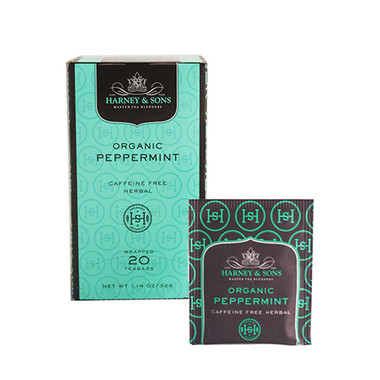Quantity Decrease Quantity Increase Quantity
•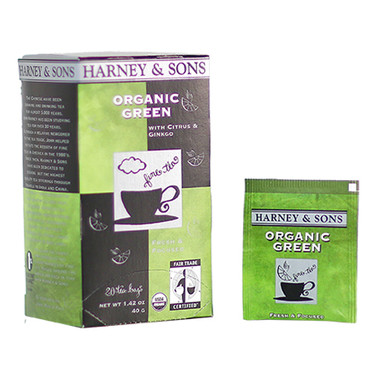Quantity Decrease Quantity Increase Quantity
•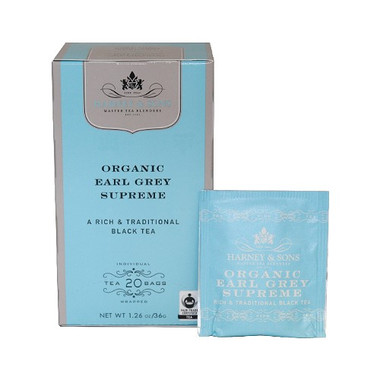Quantity Decrease Quantity Increase Quantity
•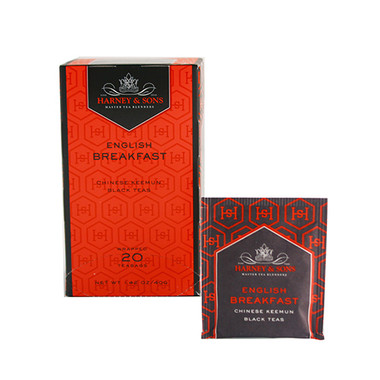Quantity Decrease Quantity Increase Quantity
•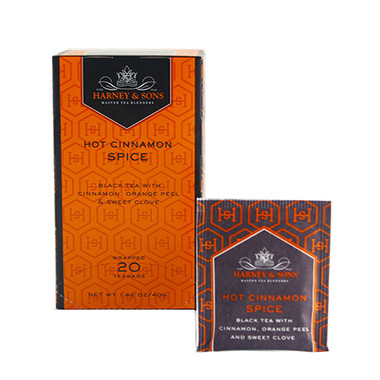Quantity Decrease Quantity Increase Quantity
•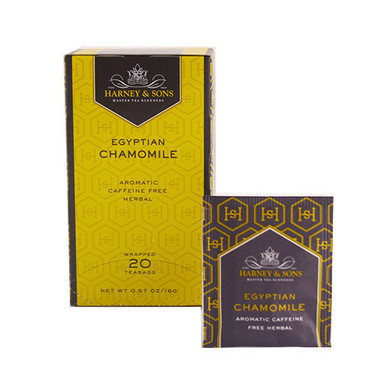Quantity Decrease Quantity Increase Quantity
•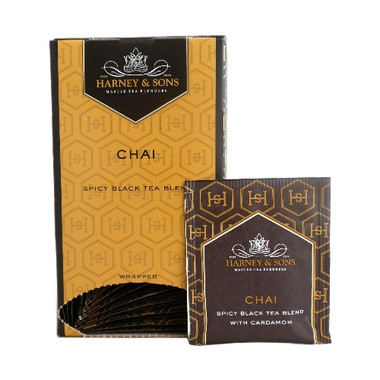Quantity Decrease Quantity Increase Quantity